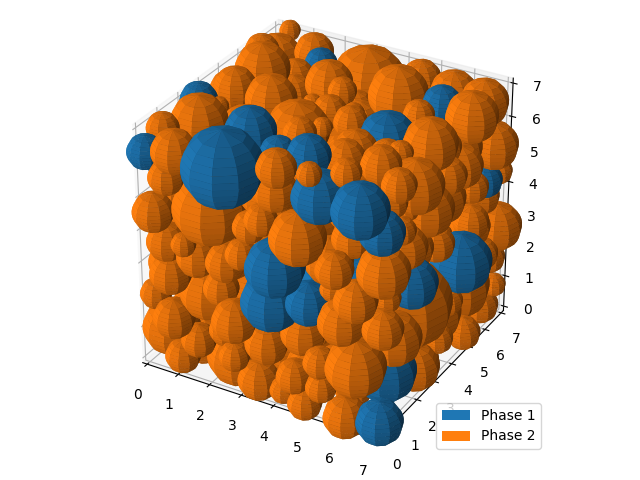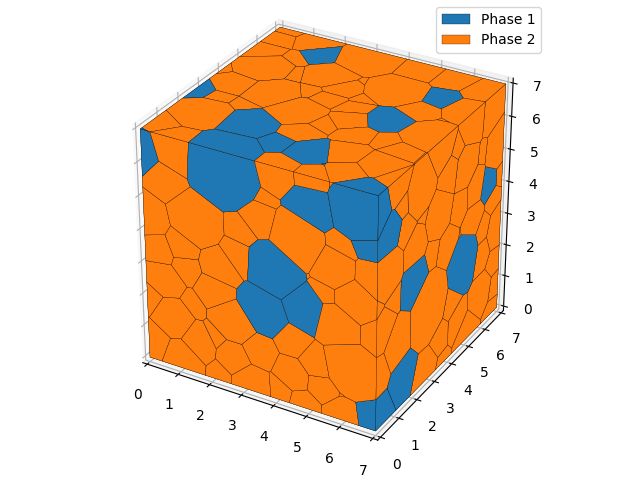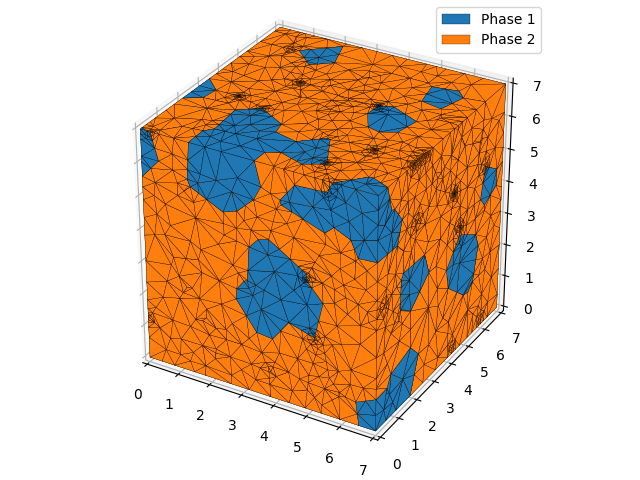# Two Phase 3D Example¶

## XML Input File¶

The basename for this file is `two_phase_3D.xml`. The file can be run using this command:

```microstructpy --demo=two_phase_3D.xml
```

The full text of the file is:

```<?xml version="1.0" encoding="UTF-8"?>
<input>
<material>
<fraction> 1 </fraction>
<volume>
<dist_type> lognorm </dist_type>
<scale> 1 </scale>
<s> 0.95 </s>
</volume>
<shape> sphere </shape>
<name> Phase 1 </name>
</material>

<material>
<fraction> 3 </fraction>
<volume>
<dist_type> lognorm </dist_type>
<scale> 0.5 </scale>
<s> 1.01 </s>
</volume>
<name> Phase 2 </name>
</material>

<domain>
<shape> cube </shape>
<side_length> 7 </side_length>
<corner> (0, 0, 0) </corner>
</domain>

<settings>
<directory> two_phase_3D </directory>
<verbose> True </verbose>

<seeds_kwargs>
<linewidths> 0.2 </linewidths>
</seeds_kwargs>
<poly_kwargs>
<linewidths> 0.2 </linewidths>
</poly_kwargs>
<tri_kwargs>
<linewidths> 0.2 </linewidths>
</tri_kwargs>
</settings>
</input>
```

## Materials¶

The first material makes up 25% of the volume, with a lognormal grain volume distribution.

The second material makes up 75% of the volume, with an independent grain volume distribution.

## Domain Geometry¶

These two materials fill a cube domain of side length 7.

## Settings¶

The function will output plots of the microstructure process and those plots are saved as PNGs. They are saved in a folder named `two_phase_3D`, in the current directory (i.e `./two_phase_3D`).

The line width of the output plots is reduced to 0.2, to make them more visible.

## Output Files¶

The three plots that this file generates are the seeding, the polygon mesh, and the triangular mesh. These three plots are shown in Fig. 14 - Fig. 16.Fig. 14 Two phase 3D example - seed geometries.¶Fig. 15 Two phase 3D example - polygonal mesh.¶Fig. 16 Two phase 3D example - triangular mesh.¶